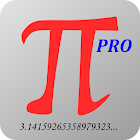MathPro mathematics all levels

All Android applications categories

All Android games categories# MathPro mathematics all levels

by: 18 8.4

8.4 Users
rating

## Screenshots

Description

Math on the phone!

Now you have all useful math formulas and definitions handy and can always refer to them. There are a lot of drawings (including 3D), explaining all symbols used in formulas.
Range of material - high school and University.

The content is divided into sections and subsections so that it is easy to find any formula, definition or equation.

There is also a free version of MathPro, which displays ads.

Algebra
- Constants
- Basics
- Exponents
- Averages
- Polynomials
- Logarithms
- Equations
- Equation systems
- Matrices
- Determinants
Analysis
- Sequences
- Limits
- Series
- Integrals, properties
- Integrals, basic formulas
- Integrals of rational functions
- Integrals of irrational functions
- Integrals of logarithmic functions
- Integrals of exponential functions
- Integrals of sine functions
Geometry
- Triangles
- Trigonometry
- Polygons
- Circles
- Polyhedrons
- Solids of revolution
- Platonic Solids
Statistics
- Combinatorics
- Probability
Logic, Sets
- Logic

Coming soon: vector calculus, differential calculus, analytic geometry, complex numbers, ... then the next sections.

from 18 reviews

"Great"

8.4